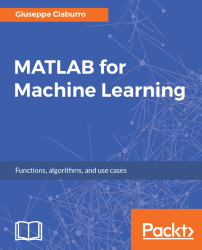•#### MATLAB for Machine Learning#### Overview of this book

MATLAB is the language of choice for many researchers and mathematics experts for machine learning. This book will help you build a foundation in machine learning using MATLAB for beginners. You’ll start by getting your system ready with t he MATLAB environment for machine learning and you’ll see how to easily interact with the Matlab workspace. We’ll then move on to data cleansing, mining and analyzing various data types in machine learning and you’ll see how to display data values on a plot. Next, you’ll get to know about the different types of regression techniques and how to apply them to your data using the MATLAB functions. You’ll understand the basic concepts of neural networks and perform data fitting, pattern recognition, and clustering analysis. Finally, you’ll explore feature selection and extraction techniques for dimensionality reduction for performance improvement. At the end of the book, you will learn to put it all together into real-world cases covering major machine learning algorithms and be comfortable in performing machine learning with MATLAB.
Title PageCreditsForewordwww.PacktPub.comCustomer FeedbackPrefaceFree Chapter
Getting Started with MATLAB Machine LearningImporting and Organizing Data in MATLABFrom Data to Knowledge DiscoveryFinding Relationships between Variables - Regression TechniquesPattern Recognition through Classification AlgorithmsIdentifying Groups of Data Using Clustering MethodsSimulation of Human Thinking - Artificial Neural NetworksImproving the Performance of the Machine Learning Model - Dimensionality ReductionMachine Learning in Practice## Summary

In this chapter, we began exploring the different types of variables: quantitative (interval and ratio) and qualitative variables (nominal, dichotomous, and ordinal). Then, we started the hard work of data preparation; we saw how to find missing values, change the datatype, replace missing values, remove missing entries, order the table, find outliers, and finally, organize multiple sources of data into one.

Next, we discovered the exploratory statistics techniques used to derive features that can guide us in choosing the right tools to extract knowledge from data. We took a look at measures of location such as mean, median, mode, quantiles, and percentiles; measures of dispersion such as range, interquartile range, variance, standard deviation, correlation, and covariance; and measures of shape such as skewness and kurtosis.

Finally, we discussed exploratory visualization to identify specific trends through a visual inspection of data. We learned how to draw histograms, box plots...